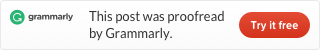# How to calculate today’s date in Excel, VBA code in Excel, DAX, Power Query, R, PowerShell, CMD and SQL

No matter what kind of tool you use need to get today’s date remains.
Here is how to do that in eight different environments.Excel and DAX

In Excel and DAX today’s date is calculated with the same function.

`=TODAY()`

If you have to deal with date and time, then there is a quick way how to get only a date. Function NOW() represents DateTime.

`=TRUNC(NOW())`

VBA

If you have to get today’s date with VBA code in Excel, then there is a Date function. Here is VBA code where today’s date is assigned to a variable Today in your regional format.

```Sub DateToday()

Today = Date

End Sub```

Here is VBA code where today’s date is assigned to a variable Today in format MM-DD-YYYY.

```Sub DateToday()

Today = Date\$

End Sub```

Today’s date in Power Query calculations.

`=DateTime.Date(DateTime.LocalNow())`

Today’s date in R.

`Sys.Date()`

Today’s date in Windows PowerShell.

`Get-Date`

If you want a different date format, then add -Format parameter.

`Get-Date -Format o`

Today’s date in the Windows command line.

`DATE /T`

Today’s date in SQL.

`CONVERT(DATE,GETDATE())`